# Category Theory & Programming

for Rivieria Scala Clojure (Note this presentation uses Haskell)
by Yann Esposito
HTML presentation: use arrows, space, swipe to navigate.

## Plan

• General overview
• Definitions
• Applications

## General Overview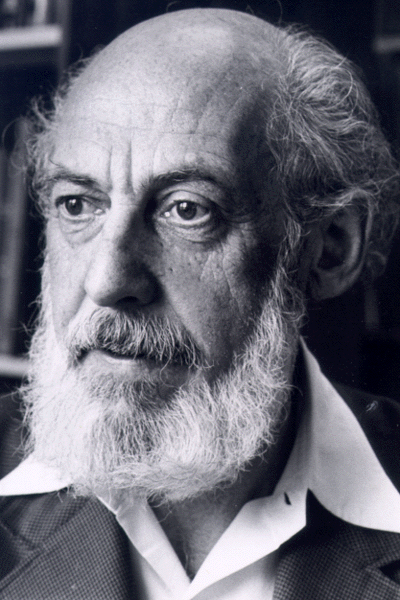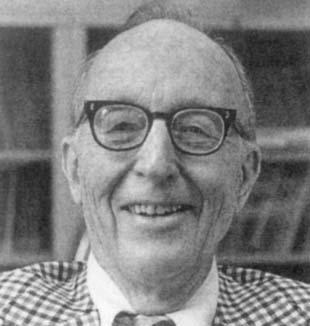Recent Math Field
1942-45, Samuel Eilenberg & Saunders Mac Lane

Certainly one of the more abstract branches of math

• New math foundation
formalism abstraction, package entire theory
• Bridge between disciplines
Physics, Quantum Physics, Topology, Logic, Computer Science

## From a Programmer perspective

Category Theory is a new language/framework for Math

• Another way of thinking
• Extremely efficient for generalization

## Math Programming relation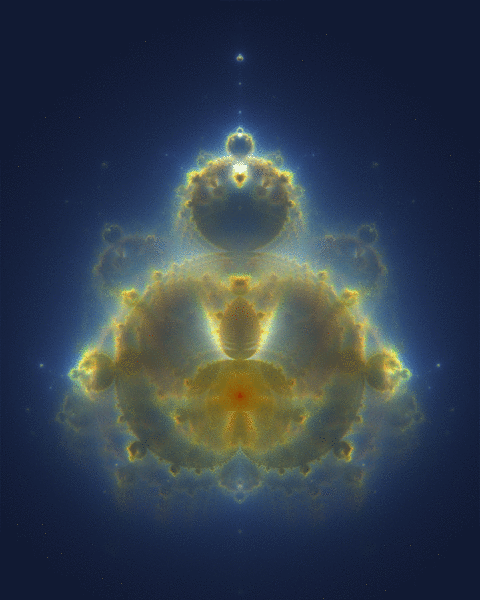Programming is doing Math

Strong relations between type theory and category theory.

Not convinced?
Certainly a vocabulary problem.

One of the goal of Category Theory is to create a homogeneous vocabulary between different disciplines.

## Vocabulary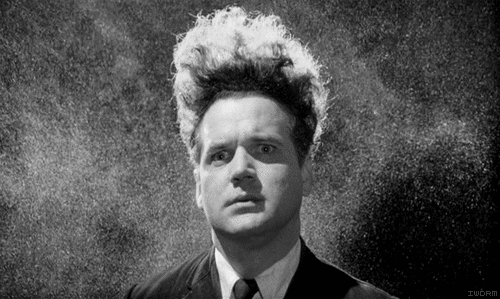Math vocabulary used in this presentation:

Category, Morphism, Associativity, Preorder, Functor, Endofunctor, Categorial property, Commutative diagram, Isomorph, Initial, Dual, Monoid, Natural transformation, Monad, Klesli arrows, κατα-morphism, ...

## Programmer TranslationMathematician Programmer
Morphism Arrow
Monoid String-like
Preorder Acyclic graph
Isomorph The same
Natural transformation rearrangement function
Funny Category LOLCat

## Plan

• General overview
• Definitions
• Category
• Intuition
• Examples
• Functor
• Examples
• Applications

## Category

A way of representing things and ways to go between things.

A Category $$\mathcal{C}$$ is defined by:

• Objects $$\ob{C}$$,
• Morphisms $$\hom{C}$$,
• a Composition law (∘)
• obeying some Properties.

## Category: Objects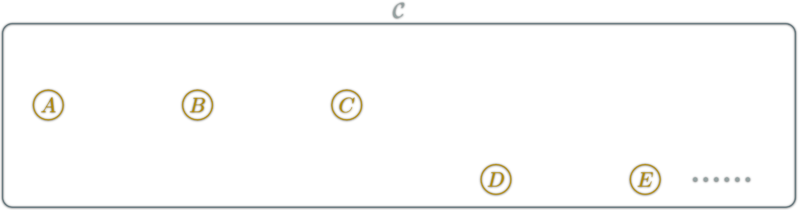$$\ob{\mathcal{C}}$$ is a collection

## Category: Morphisms$$A$$ and $$B$$ objects of $$\C$$
$$\hom{A,B}$$ is a collection of morphisms
$$f:A→B$$ denote the fact $$f$$ belongs to $$\hom{A,B}$$

$$\hom{\C}$$ the collection of all morphisms of $$\C$$

## Category: Composition

Composition (∘): associate to each couple $$f:A→B, g:B→C$$ $$g∘f:A\rightarrow C$$## Category laws: neutral element

for each object $$X$$, there is an $$\id_X:X→X$$,
such that for each $$f:A→B$$:## Category laws: Associativity

Composition is associative:## Commutative diagrams

Two path with the same source and destination are equal.$$(h∘g)∘f = h∘(g∘f)$$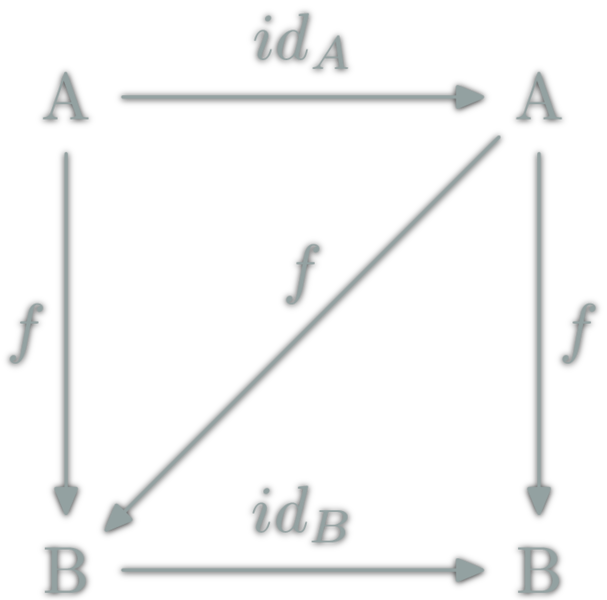$$id_B∘f = f = f∘id_A$$

## Can this be a category?

$$\ob{\C},\hom{\C}$$ fixed, is there a valid ∘?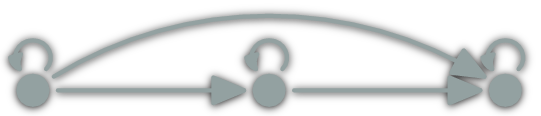YESno candidate for $$g∘f$$ NOYES

## Can this be a category?no candidate for $$f:C→B$$ NO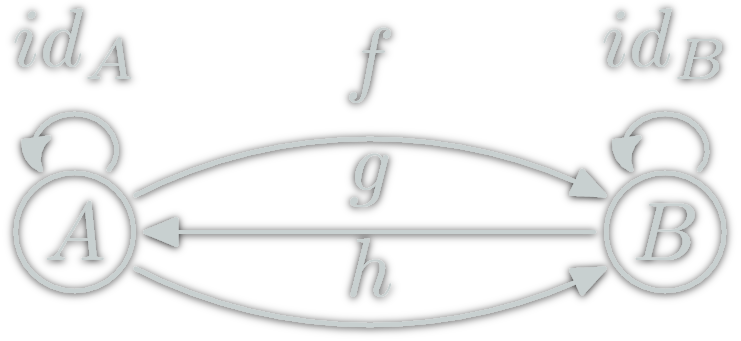$$(h∘g)∘f=\id_B∘f=f$$ $$h∘(g∘f)=h∘\id_A=h$$ but $$h≠f$$ NO

## Category $$\Set$$

• $$\ob{\Set}$$ are all the sets
• $$\hom{E,F}$$ are all functions from $$E$$ to $$F$$
• ∘ is functions composition
• $$\ob{\Set}$$ is a proper class ; not a set
• $$\hom{E,F}$$ is a set
• $$\Set$$ is then a locally small category

## Categories Everywhere?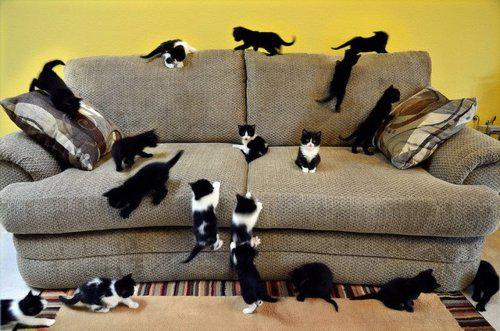• $$\Mon$$: (monoids, monoid morphisms,∘)
• $$\Vec$$: (Vectorial spaces, linear functions,∘)
• $$\Grp$$: (groups, group morphisms,∘)
• $$\Rng$$: (rings, ring morphisms,∘)
• Any deductive system T: (theorems, proofs, proof concatenation)
• $$\Hask$$: (Haskell types, functions, (.) )
• ...

## Smaller Examples

### Strings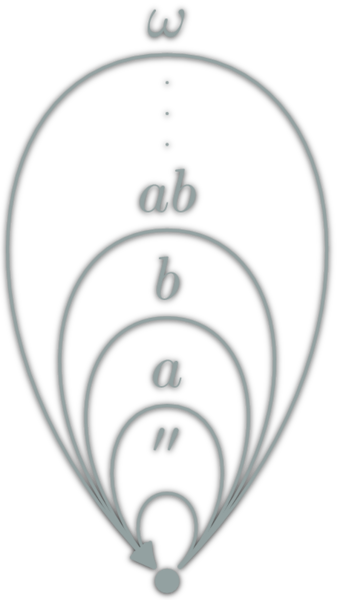• $$\ob{Str}$$ is a singleton
• $$\hom{Str}$$ each string
• ∘ is concatenation (++)
• "" ++ u = u = u ++ ""
• (u ++ v) ++ w = u ++ (v ++ w)

## Finite Example?

### Graph

• $$\ob{G}$$ are vertices
• $$\hom{G}$$ each path
• ∘ is path concatenation
• $$\ob{G}=\{X,Y,Z\}$$,
• $$\hom{G}=\{ε,α,β,γ,αβ,βγ,...\}$$
• $$αβ∘γ=αβγ$$

## Number construction

### Each Numbers as a whole category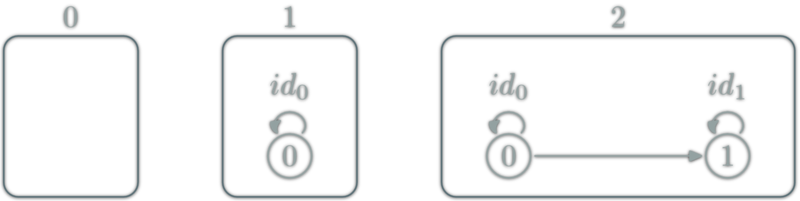## Degenerated Categories: MonoidsEach Monoid $$(M,e,⊙): \ob{M}=\{∙\},\hom{M}=M,\circ = ⊙$$

Only one object.

Examples:

• (Integer,0,+), (Integer,1,*),
• (Strings,"",++), for each a, ([a],[],++)

## Degenerated Categories: Preorders $$(P,≤)$$

• $$\ob{P}={P}$$,
• $$\hom{x,y}=\{x≤y\} ⇔ x≤y$$,
• $$(y≤z) \circ (x≤y) = (x≤z)$$

At most one morphism between two objects.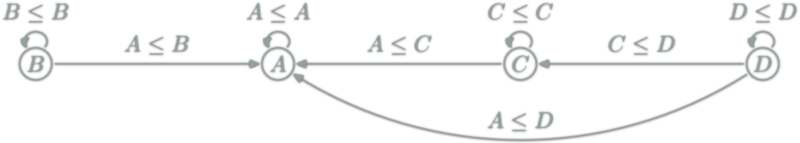## Degenerated Categories: Discrete Categories### Any Set

Any set $$E: \ob{E}=E, \hom{x,y}=\{x\} ⇔ x=y$$

Only identities

## Choice

The same object can be seen in many different way as a category.

You can choose what are object, morphisms and composition.

ex: Str and discrete(Σ*)

## Categorical Properties

Any property which can be expressed in term of category, objects, morphism and composition.

• Dual: $$\D$$ is $$\C$$ with reversed morphisms.
• Initial: $$Z\in\ob{\C}$$ s.t. $$∀Y∈\ob{\C}, \#\hom{Z,Y}=1$$
Unique ("up to isormophism")
• Terminal: $$T\in\ob{\C}$$ s.t. $$T$$ is initial in the dual of $$\C$$
• Functor: structure preserving mapping between categories
• ...

## Isomorph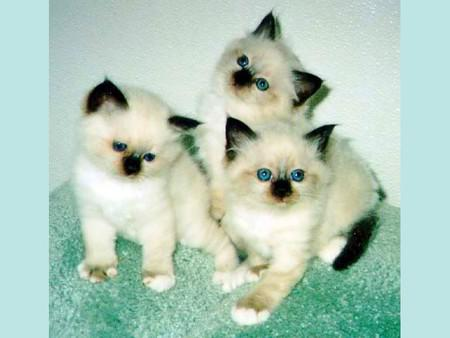isomorphism: $$f:A→B$$ which can be "undone" i.e.
$$∃g:B→A$$, $$g∘f=id_A$$ & $$f∘g=id_B$$
in this case, $$A$$ & $$B$$ are isomorphic.

A≌B means A and B are essentially the same.
In Category Theory, = is in fact mostly .
For example in commutative diagrams.

## Functor

A functor is a mapping between two categories. Let $$\C$$ and $$\D$$ be two categories. A functor $$\F$$ from $$\C$$ to $$\D$$:

• Associate objects: $$A\in\ob{\C}$$ to $$\F(A)\in\ob{\D}$$
• Associate morphisms: $$f:A\to B$$ to $$\F(f) : \F(A) \to \F(B)$$ such that
• $$\F ($$$$\id_X$$$$)=$$$$\id$$$$\vphantom{\id}_{\F(}$$$$\vphantom{\id}_X$$$$\vphantom{\id}_{)}$$,
• $$\F ($$$$g∘f$$$$)=$$$$\F($$$$g$$$$)$$$$\circ$$$$\F($$$$f$$$$)$$

## Functor Example (ob → ob)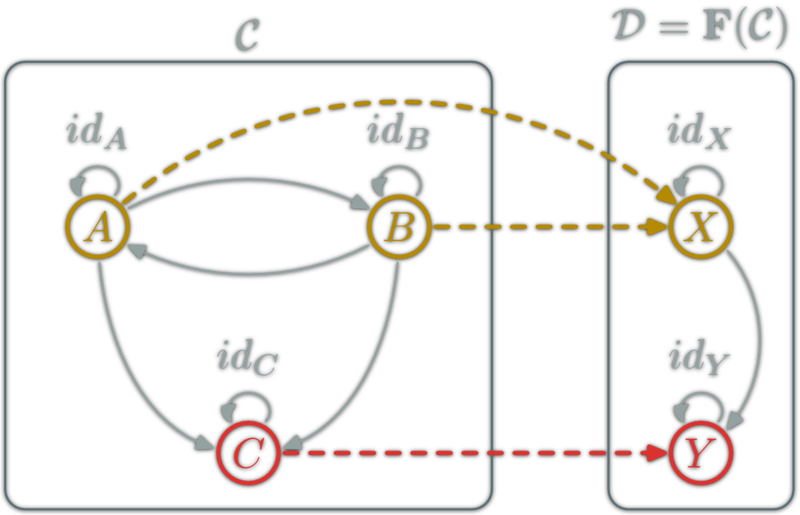## Functor Example (hom → hom)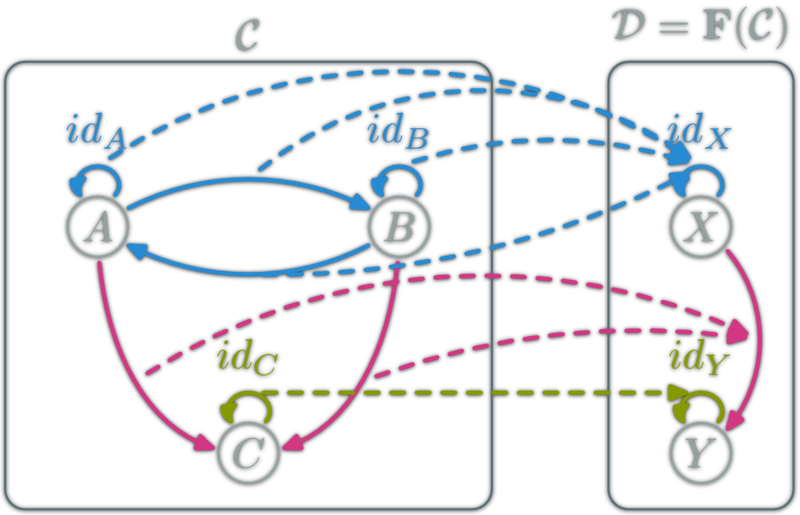## Functor Example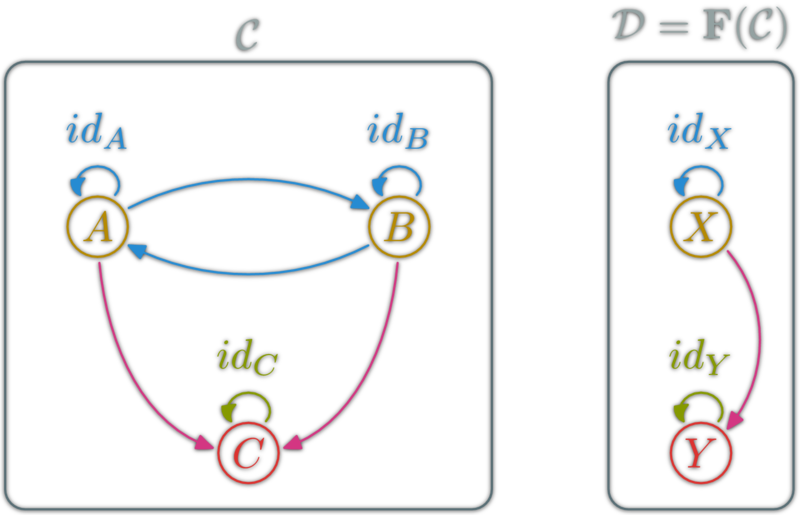## Endofunctors

An endofunctor for $$\C$$ is a functor $$F:\C→\C$$.## Category of CategoriesCategories and functors form a category: $$\Cat$$

• $$\ob{\Cat}$$ are categories
• $$\hom{\Cat}$$ are functors
• ∘ is functor composition

## Plan

• General overview
• Definitions
• Applications
• $$\Hask$$ category
• Functors
• Natural transformations
• κατα-morphisms

Category $$\Hask$$: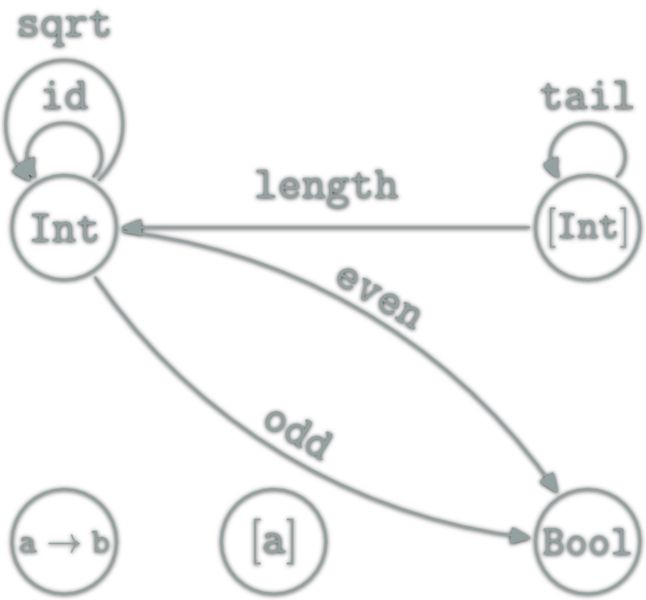• $$\ob{\Hask} =$$ Haskell types
• $$\hom{\Hask} =$$ Haskell functions
• ∘ = (.) Haskell function composition

Forget glitches because of undefined.

In Haskell some types can take type variable(s). Typically: [a].

Types have kinds; The kind is to type what type is to function. Kind are the types for types (so meta).

Int, Char :: *
[], Maybe :: * -> *
(,), (->) :: * -> * -> *
[Int], Maybe Char, Maybe [Int] :: *

Sometimes, the type determine a lot about the function:

fst :: (a,b) -> a -- Only one choice
snd :: (a,b) -> b -- Only one choice
f :: a -> [a]     -- Many choices
-- Possibilities: f x=[], or [x], or [x,x] or [x,...,x]

? :: [a] -> [a] -- Many choices
-- can only rearrange: duplicate/remove/reorder elements
-- for example: the type of addOne isn't [a] -> [a]
addOne l = map (+1) l
-- The (+1) force 'a' to be a Num.

## Haskell Functor vs $$\Hask$$ Functor

A Haskell Functor is a type F :: * -> * which belong to the type class Functor ; thus instantiate fmap :: (a -> b) -> (F a -> F b).

& F: $$\ob{\Hask}→\ob{\Hask}$$
& fmap: $$\hom{\Hask}→\hom{\Hask}$$

The couple (F,fmap) is a $$\Hask$$'s functor if for any x :: F a:

• fmap id x = x
• fmap (f.g) x= (fmap f . fmap g) x

## Haskell Functors Example: Maybe

data Maybe a = Just a | Nothing
instance Functor Maybe where
fmap :: (a -> b) -> (Maybe a -> Maybe b)
fmap f (Just a) = Just (f a)
fmap f Nothing = Nothing
fmap (+1) (Just 1) == Just 2
fmap (+1) Nothing  == Nothing
fmap head (Just [1,2,3]) == Just 1

## Haskell Functors Example: List

instance Functor ([]) where
fmap :: (a -> b) -> [a] -> [b]
fmap = map
fmap (+1) [1,2,3]           == [2,3,4]
fmap (+1) []                == []
fmap head [[1,2,3],[4,5,6]] == [1,4]

## Haskell Functors for the programmer

Functor is a type class used for types that can be mapped over.

• Containers: [], Trees, Map, HashMap...
• "Feature Type":
• Maybe a: help to handle absence of a.
Ex: safeDiv x 0 ⇒ Nothing
• Either String a: help to handle errors
Ex: reportDiv x 0 ⇒ Left "Division by 0!"

## Haskell Functor intuition

Put normal function inside a container. Ex: list, trees...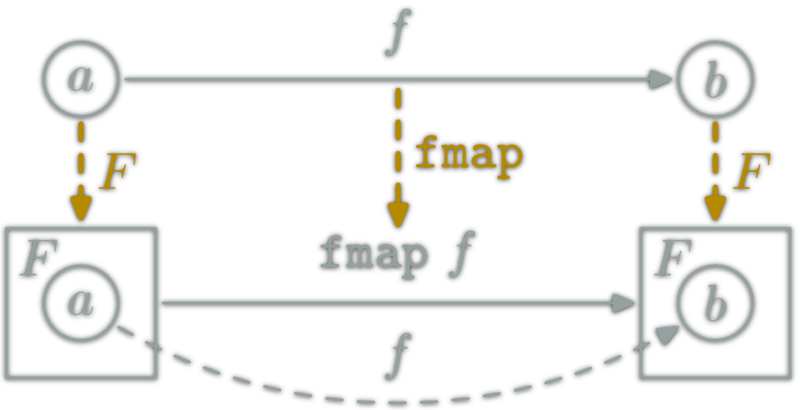## Haskell Functor properties

• endofunctors ; $$F:\C→\C$$ here $$\C = \Hask$$,
• a couple (Object,Morphism) in $$\Hask$$.

## Functor as boxes

Haskell functor can be seen as boxes containing all Haskell types and functions. Haskell types is fractal:## Functor as boxes

Haskell functor can be seen as boxes containing all Haskell types and functions. Haskell types is fractal: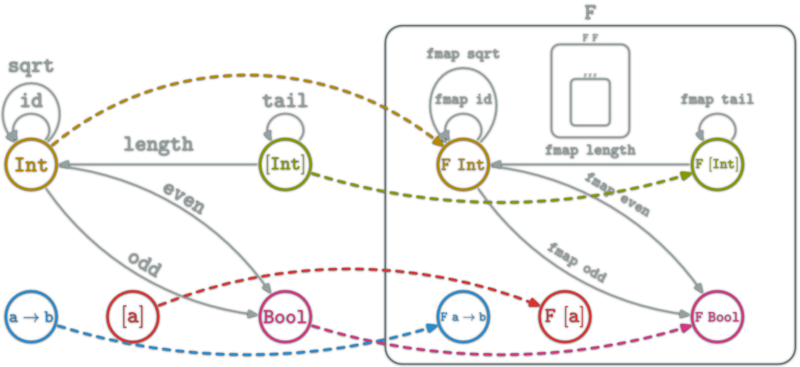## Functor as boxes

Haskell functor can be seen as boxes containing all Haskell types and functions. Haskell types is fractal:A simple basic example is the $$id_\Hask$$ functor. It simply cannot be expressed as a couple (F,fmap) where

• F::* -> *
• fmap :: (a -> b) -> (F a) -> (F b)

Another example:

• F(T)=Int
• F(f)=\_->0

## Also Functor inside $$\Hask$$

$$\mathtt{[a]}∈\ob{\Hask}$$ but is also a category. Idem for Int.

length is a Functor from the category [a] to the category Int:

• $$\ob{\mathtt{[a]}}=\{∙\}$$
• $$\hom{\mathtt{[a]}}=\mathtt{[a]}$$
• $$∘=\mathtt{(++)}$$

• $$\ob{\mathtt{Int}}=\{∙\}$$
• $$\hom{\mathtt{Int}}=\mathtt{Int}$$
• $$∘=\mathtt{(+)}$$
• id: length [] = 0
• comp: length (l ++ l') = (length l) + (length l')

## Category of $$\Hask$$ Endofunctors## Category of Functors

If $$\C$$ is small ($$\hom{\C}$$ is a set). All functors from $$\C$$ to some category $$\D$$ form the category $$\mathrm{Func}(\C,\D)$$.

• $$\ob{\mathrm{Func}(\C,\D)}$$: Functors $$F:\C→\D$$
• $$\hom{\mathrm{Func}(\C,\D)}$$: natural transformations
• ∘: Functor composition

$$\mathrm{Func}(\C,\C)$$ is the category of endofunctors of $$\C$$.

## Natural Transformations

Let $$F$$ and $$G$$ be two functors from $$\C$$ to $$\D$$.A natural transformation: familly η ; $$η_X\in\hom{\D}$$ for $$X\in\ob{\C}$$ s.t.

ex: between Haskell functors; F a -> G a
Rearragement functions only.

## Natural Transformation Examples (1/4)

data List a = Nil | Cons a (List a)
toList :: [a] -> List a
toList [] = Nil
toList (x:xs) = Cons x (toList xs)
 

toList is a natural transformation. It is also a morphism from [] to List in the Category of $$\Hask$$ endofunctors.## Natural Transformation Examples (2/4)

data List a = Nil | Cons a (List a)
toHList :: List a -> [a]
toHList Nil = []
toHList (Cons x xs) = x:toHList xs
 

toHList is a natural transformation. It is also a morphism from List to [] in the Category of $$\Hask$$ endofunctors.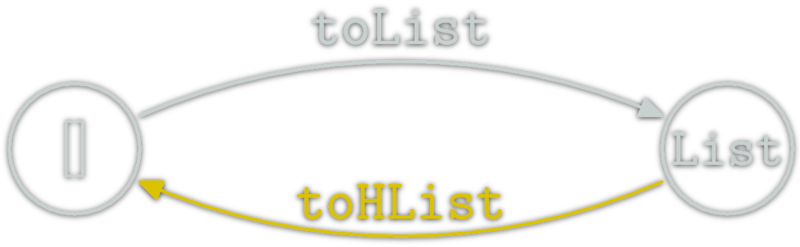toList . toHList = id & toHList . toList = id & therefore [] & List are isomorph.

## Natural Transformation Examples (3/4)

toMaybe :: [a] -> Maybe a
toMaybe [] = Nothing
toMaybe (x:xs) = Just x
 

toMaybe is a natural transformation. It is also a morphism from [] to Maybe in the Category of $$\Hask$$ endofunctors.## Natural Transformation Examples (4/4)

mToList :: Maybe a -> [a]
mToList Nothing = []
mToList Just x  = [x]
 

toMaybe is a natural transformation. It is also a morphism from [] to Maybe in the Category of $$\Hask$$ endofunctors.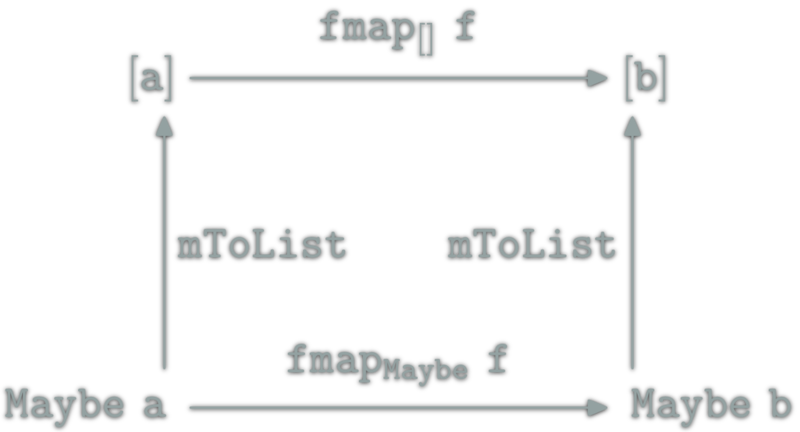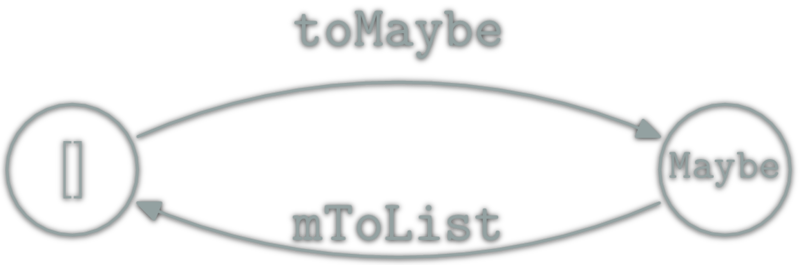There is no isomorphism. Hint: Bool lists longer than 1.

## Composition problem

The Problem; example with lists:

f x = [x]       ⇒ f 1 =    ⇒ (f.f) 1 = [] ✗
g x = [x+1]     ⇒ g 1 =    ⇒ (g.g) 1 = ERROR +1 ✗
h x = [x+1,x*3] ⇒ h 1 = [2,3] ⇒ (h.h) 1 = ERROR [2,3]+1 ✗ 

The same problem with most f :: a -> F a functions and functor F.

## Composition Fixable?

How to fix that? We want to construct an operator which is able to compose:

f :: a -> F b & g :: b -> F c.

More specifically we want to create an operator ◎ of type

◎ :: (b -> F c) -> (a -> F b) -> (a -> F c)

Note: if F = I, ◎ = (.).

## Fix Composition (1/2)

Goal, find: ◎ :: (b -> F c) -> (a -> F b) -> (a -> F c)
f :: a -> F b, g :: b -> F c:

• (g ◎ f) x ???
• First apply f to xf x :: F b
• Then how to apply g properly to an element of type F b?

## Fix Composition (2/2)

Goal, find: ◎ :: (b -> F c) -> (a -> F b) -> (a -> F c)
f :: a -> F b, g :: b -> F c, f x :: F b:

• Use fmap :: (t -> u) -> (F t -> F u)!
• (fmap g) :: F b -> F (F c) ; (t=b, u=F c)
• (fmap g) (f x) :: F (F c) it almost WORKS!
• We lack an important component, join :: F (F c) -> F c
• (g ◎ f) x = join ((fmap g) (f x))
◎ is the Kleisli composition; in Haskell: <=< (in Control.Monad).

## Necessary laws

For ◎ to work like composition, we need join to hold the following properties:

• join (join (F (F (F a))))=join (F (join (F (F a))))
• abusing notations denoting join by ⊙; this is equivalent to
(F ⊙ F) ⊙ F = F ⊙ (F ⊙ F)
• There exists η :: a -> F a s.t.
η⊙F=F=F⊙η

## Klesli composition

Now the composition works as expected. In Haskell ◎ is <=< in Control.Monad.

g <=< f = \x -> join ((fmap g) (f x))

f x = [x]       ⇒ f 1 =    ⇒ (f <=< f) 1 =  ✓
g x = [x+1]     ⇒ g 1 =    ⇒ (g <=< g) 1 =  ✓
h x = [x+1,x*3] ⇒ h 1 = [2,3] ⇒ (h <=< h) 1 = [3,6,4,9] ✓

## We reinvented Monads!

A monad is a triplet (M,⊙,η) where

• $$M$$ an Endofunctor (to type a associate M a)
• $$⊙:M×M→M$$ a nat. trans. (i.e. ⊙::M (M a) → M a ; join)
• $$η:I→M$$ a nat. trans. ($$I$$ identity functor ; η::a → M a)

Satisfying

• $$M ⊙ (M ⊙ M) = (M ⊙ M) ⊙ M$$
• $$η ⊙ M = M = M ⊙ η$$

## Compare with Monoid

A Monoid is a triplet $$(E,∙,e)$$ s.t.

• $$E$$ a set
• $$∙:E×E→E$$
• $$e:1→E$$

Satisfying

• $$x∙(y∙z) = (x∙y)∙z, ∀x,y,z∈E$$
• $$e∙x = x = x∙e, ∀x∈E$$

## Monads are just Monoids

A Monad is just a monoid in the category of endofunctors, what's the problem?

The real sentence was:

All told, a monad in X is just a monoid in the category of endofunctors of X, with product × replaced by composition of endofunctors and unit set by the identity endofunctor.

## Example: List

• [] :: * -> * an Endofunctor
• $$⊙:M×M→M$$ a nat. trans. (join :: M (M a) -> M a)
• $$η:I→M$$ a nat. trans.
-- In Haskell ⊙ is "join" in "Control.Monad"
join :: [[a]] -> [a]
join = concat

-- In Haskell the "return" function (unfortunate name)
η :: a -> [a]
η x = [x]

## Example: List (law verification)

Example: List is a functor (join is ⊙)

• $$M ⊙ (M ⊙ M) = (M ⊙ M) ⊙ M$$
• $$η ⊙ M = M = M ⊙ η$$
join [ join [[x,y,...,z]] ] = join [[x,y,...,z]]
= join (join [[[x,y,...,z]]])
join (η [x]) = [x] = join [η x]

Therefore ([],join,η) is a monad.

A LOT of monad tutorial on the net. Just one example; the State Monad

DrawScene to State Screen DrawScene ; still pure.

main = drawImage (width,height)

drawImage :: Screen -> DrawScene
drawImage screen = do
drawPoint p screen
drawCircle c screen
drawRectangle r screen

drawPoint point screen = ...
drawCircle circle screen = ...
drawRectangle rectangle screen = ...
main = do
put (Screen 1024 768)
drawImage

drawImage :: State Screen DrawScene
drawImage = do
drawPoint p
drawCircle c
drawRectangle r

drawPoint :: Point -> State Screen DrawScene
drawPoint p = do
Screen width height <- get
...

## fold## κατα-morphism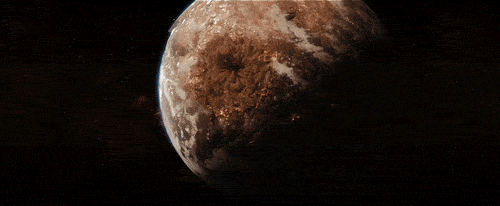## κατα-morphism: fold generalization

acc type of the "accumulator":
fold :: (acc -> a -> acc) -> acc -> [a] -> acc

Idea: put the accumulated value inside the type.

-- Equivalent to fold (+1) 0 "cata"
(Cons 'c' (Cons 'a' (Cons 't' (Cons 'a' Nil))))
(Cons 'c' (Cons 'a' (Cons 't' (Cons 'a' 0))))
(Cons 'c' (Cons 'a' (Cons 't' 1)))
(Cons 'c' (Cons 'a' 2))
(Cons 'c' 3)
4

But where are all the informations? (+1) and 0?

## κατα-morphism: Missing Information

Where is the missing information?

• Functor operator fmap
• Algebra representing the (+1) and also knowing about the 0.

First example, make length on [Char]

## κατα-morphism: Type work


data StrF a = Cons Char a | Nil
data Str' = StrF Str'

-- generalize the construction of Str to other datatype
-- Mu: type fixed point
-- Mu :: (* -> *) -> *

data Mu f = InF { outF :: f (Mu f) }
data Str = Mu StrF

-- Example
foo=InF { outF = Cons 'f'
(InF { outF = Cons 'o'
(InF { outF = Cons 'o'
(InF { outF = Nil })})})}

## κατα-morphism: missing information retrieved

type Algebra f a = f a -> a
instance Functor (StrF a) =
fmap f (Cons c x) = Cons c (f x)
fmap _ Nil = Nil
cata :: Functor f => Algebra f a -> Mu f -> a
cata f = f . fmap (cata f) . outF

## κατα-morphism: Finally length

All needed information for making length.

instance Functor (StrF a) =
fmap f (Cons c x) = Cons c (f x)
fmap _ Nil = Nil

length' :: Str -> Int
length' = cata phi where
phi :: Algebra StrF Int -- StrF Int -> Int
phi (Cons a b) = 1 + b
phi Nil = 0

main = do
l <- length' \$ stringToStr "Toto"
...

## κατα-morphism: extension to Trees

Once you get the trick, it is easy to extent to most Functor.

type Tree = Mu TreeF
data TreeF x = Node Int [x]

instance Functor TreeF where
fmap f (Node e xs) = Node e (fmap f xs)

depth = cata phi where
phi :: Algebra TreeF Int -- TreeF Int -> Int
phi (Node x sons) = 1 + foldr max 0 sons

## Conclusion

Category Theory oriented Programming:

• Focus on the type and operators
• Extreme generalisation
• Better modularity
• Better control through properties of types

No cat were harmed in the making of this presentation.

/

#